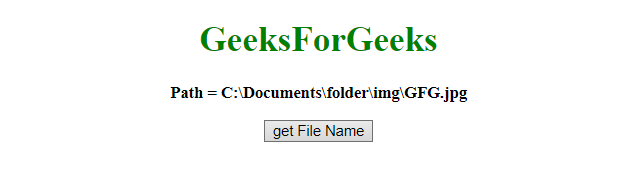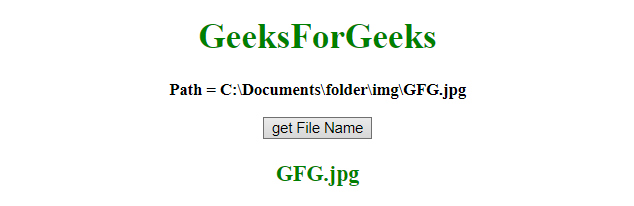Related Articles
How to get the file name from full path using JavaScript ?
• Last Updated : 10 Jun, 2019

Given a file name which contains the file path also, the task is to get the file name from full path. There are a few methods to solve this problem which are listed below:

• replace() method: This method searches a string for a defined value, or a regular expression, and returns a new string with the replaced defined value.

Syntax:

`string.replace(searchVal, newvalue)`

Parameters: This method accepts two parameters as mentioned above and described below:

• searchVal: This parameter is required. It specifies the value, or regular expression, that is going to replace by the new value.
• newvalue: This parameter is required. It specifies the value to be replaced with the search value.

Return value: It returns a new string where the defines value(s) has been replaced by the new value.

• split() method: This method is used to split a string into an array of substrings, and returns the new array.

Syntax:

`string.split(separator, limit)`

Parameters: This method accepts two parameters as mentioned above and described below:

• separator: This parameter is optional. It specifies the character, or the regular expression, to use for splitting the string. If not used, the whole string will be returned (an array with only one item).
• limit: This parameter is optional. It specifies the integer that specifies the number of splits, items beyond the split limit will be excluded from the array.

Return value: It returns a new Array, having the splitted items.

• JavaScript Array pop() Method: This method deletes the last element of an array, and returns deleted element.

Syntax:

`array.pop()`

Return value: It returns any type, representing the deleted array item. This item can be a string, number, array, boolean, or any other object types which are allowed in an array.

Example 1: This example gets the file name with the help of Regular Expression by using replace() method.

 ` ``<``html``> ``    ``<``head``> ``        ``<``title``> ``            ``How to get the file name from a``            ``full path using JavaScript``        ````    `` ``     ` `    ``<``body` `style` `= ``"text-align:center;"``> ``         ` `        ``<``h1` `style` `= ``"color:green;"` `> ``            ``GeeksForGeeks ``        ````         ` `        ``<``p` `id` `= ``"GFG_UP"` `style` `=``            ``"font-size: 15px; font-weight: bold;"``>``        ````         ` `        ``<``button` `onclick` `= ``"gfg_Run()"``> ``            ``get File Name``        ````         ` `        ``<``p` `id` `= ``"GFG_DOWN"` `style` `= ``            ``"color:green; font-size: 20px; font-weight: bold;"``>``        ````         ` `        ``<``script``>``            ``var el_up = document.getElementById("GFG_UP");``            ``var el_down = document.getElementById("GFG_DOWN");``            ``var path = "Path = " +``                    ``"C:\\Documents\\folder\\img\\GFG.jpg";``             ` `            ``el_up.innerHTML = path;``         ` `            ``function gfg_Run() {``                ``el_down.innerHTML = path.replace(/^.*[\\\/]/, '');``            ``}         ``        `` ``    `` ```

Output:

• Before clicking on the button:• After clicking on the button:Example 2: This example gets the file name with the help of repeated split() and pop() method.

 ` ``<``html``> ``    ``<``head``> ``        ``<``title``> ``            ``How to get the file name from a``            ``full path using JavaScript``        ````    `` ``     ` `    ``<``body` `style` `= ``"text-align:center;"``> ``         ` `        ``<``h1` `style` `= ``"color:green;"` `> ``            ``GeeksForGeeks ``        ````         ` `        ``<``p` `id` `= ``"GFG_UP"` `style` `= ``            ``"font-size: 15px; font-weight: bold;"``>``        ````         ` `        ``<``button` `onclick` `= ``"gfg_Run()"``> ``            ``get File Name``        ````         ` `        ``<``p` `id` `= ``"GFG_DOWN"` `style` `=``            ``"color:green; font-size: 20px; font-weight: bold;"``>``        ````         ` `        ``<``script``>``            ``var el_up = document.getElementById("GFG_UP");``            ``var el_down = document.getElementById("GFG_DOWN");``            ``var path = "Path = " + ``                    ``"C:\\Documents\\folder\\img\\GFG.jpg";``            ``el_up.innerHTML = path;``         ` `            ``function gfg_Run() {``                ``el_down.innerHTML``                    ``= path.split('\\').pop().split('/').pop();``            ``}         ``        `` ``    `` ``                    `

Output:

• Before clicking on the button:• After clicking on the button:My Personal Notes arrow_drop_up